Next: Supersonic Compression by Turning Up: Two-Dimensional Compressible Inviscid Flow Previous: Supersonic Flow in Corner

# Weak Oblique Shocks

For small deflection angles,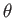, the oblique shock equations reduce to relatively simple expressions. In fact, we already saw in Equation (15.15) that if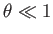then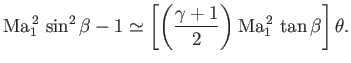(15.16)

It follows that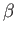is either close to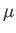or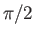, depending on whether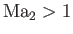or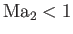. (See Figure 15.2.) For the present, we are only considering the former case (), for which we may use the approximation [see Equation (15.6)]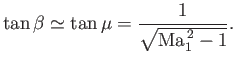(15.17)

Equation (15.16) then reduces to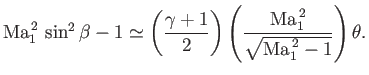(15.18)

This is the basic relation that is needed to obtain all other approximate expressions, because these expressions only depend on the normal component of the upstream flow,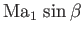. Thus, Equation (15.1) yields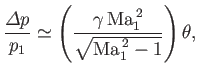(15.19)

where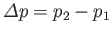. It is apparent that the shock strength,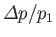, is directly proportional to the deflection angle,.

The changes in the other flow quantities, except the specific entropy, across the shock front are also directly proportional to. (See Exercise iii.) The change in the entropy, on the other hand, is proportional to the third power of the shock strength (see Section 14.8), and, hence, to the third power of the deflection angle.

To explicitly find the deviation of the wave angle,, from the Mach angle,, we write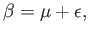(15.20)

where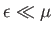. Thus, we have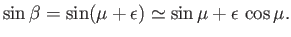(15.21)

However, by definition,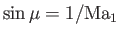, and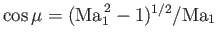[see Equation (15.6)], so we obtain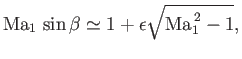(15.22)

or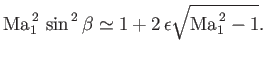(15.23)

Finally, comparison with Equation (15.18) reveals that(15.24)

In other words, for a finite deflection angle,, the wave angle,, differs from the Mach angle,, by an amount,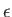, that is of the same order of magnitude as.

The change in flow speed across the shock front is obtained from the ratio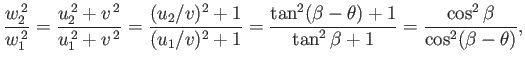(15.25)

where use has been made of Equations (15.8) and (15.9). However,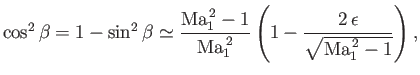(15.26)

where use has been made of Equation (15.23). Similarly,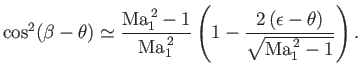(15.27)

Hence, to first order in,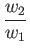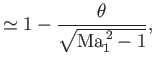(15.28)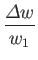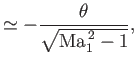(15.29)

where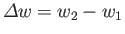.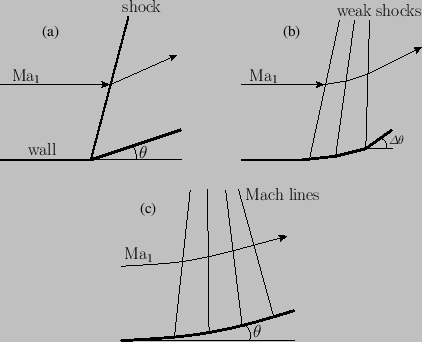Next: Supersonic Compression by Turning Up: Two-Dimensional Compressible Inviscid Flow Previous: Supersonic Flow in Corner
Richard Fitzpatrick 2016-03-31